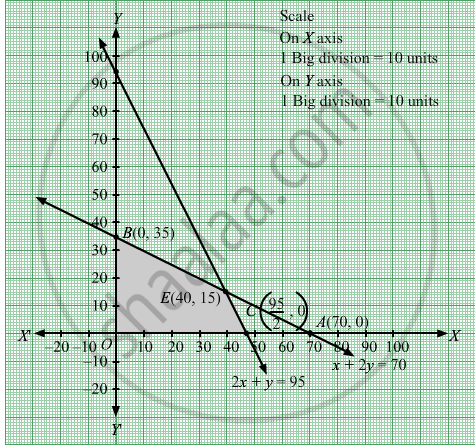# The Point at Which the Maximum Value of X + Y, Subject to the Constraints X + 2y ≤ 70, 2x + Y ≤ 95, X, Y ≥ 0 is Obtained, is (A) (30, 25) (B) (20, 35) (C) (35, 20) (D) (40, 15) - Mathematics

MCQ

The point at which the maximum value of x + y, subject to the constraints x + 2y ≤ 70, 2xy ≤ 95, xy ≥ 0 is obtained, is

•  (30, 25)

•  (20, 35)

•  (35, 20)

•  (40, 15)

#### Solution

(40, 15)

We need to maximize the function Z = y
Converting the given inequations into equations, we obtain  $x + 2y = 70, 2x + y = 95, x = 0z and y = 0$

Region represented by + 2y ≤ 70:
The line x + 2y = 70 meets the coordinate axes at A(70, 0) and B(0, 35) respectively. By joining these points we obtain the line x + 2y = 70. Clearly (0,0) satisfies the inequation + 2y ≤ 70. So,the region containing the origin represents the solution set of the inequation x + 2y ≤ 70.

Region represented by 2x + y ≤ 95:
The line 2x y = 95 meets the coordinate axes at $C\left( \frac{95}{2}, 0 \right)$  respectively. By joining these points we obtain the line 2x y = 95.

Clearly (0,0) satisfies the inequation 2x + y ≤ 95. So,the region containing the origin represents the solution set of the inequation 2x + y ≤ 95.

Region represented by x ≥ 0 and y ≥ 0:
Since, every point in the first quadrant satisfies these inequations. So, the first quadrant is the region represented by the inequations x ≥ 0, and ≥ 0.

The feasible region determined by the system of constraints + 2y ≤ 70, 2x + y ≤ 95, x ≥ 0, and y ≥ 0, are as follows.The corner points of the feasible region are O(0, 0), $C\left( \frac{95}{2}, 0 \right)$,E ( 40 , 15) and B (0 , 35 ) .

The values of Z at these corner points are as follows.

 Corner point Z = x + y O(0, 0) 0 + 0 = 0 $C\left( \frac{95}{2}, 0 \right)$ $\frac{95}{2}$ +0 =  $\frac{95}{2}$ $E\left( 40, 15 \right)$ 40 +15 = 55 B(0, 35) 0 + 35 = 35

We see that the maximum value of the objective function Z is 55 which is at  $\left( 40, 15 \right)$ .
Concept: Introduction of Linear Programming
Is there an error in this question or solution?

#### APPEARS IN

RD Sharma Class 12 Maths
Chapter 30 Linear programming
MCQ | Q 15 | Page 68